## Example Questions

### Example Question #5 : How To Find Out If Lines Are Parallel

Which of the following lines is parallel to: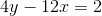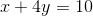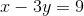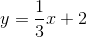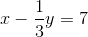Explanation:

First write the equation in slope intercept form. Add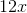to both sides to get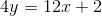. Now divide both sides byto get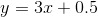. The slope of this line is, so any line that also has a slope ofwould be parallel to it. The correct answer is.

### Example Question #7 : How To Find Out If Lines Are Parallel

Which pair of linear equations represent parallel lines?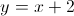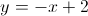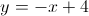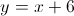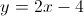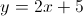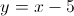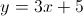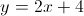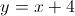Explanation:

Parallel lines will always have equal slopes. The slope can be found quickly by observing the equation in slope-intercept form and seeing which number falls in the "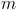" spot in the linear equation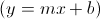We are looking for an answer choice in which both equations have the samevalue. Both lines in the correct answer have a slope of 2, therefore they are parallel.

### Example Question #8 : How To Find Out If Lines Are Parallel

Which of the following equations represents a line that is parallel to the line represented by the equation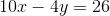?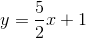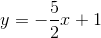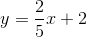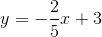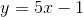Explanation:

Lines are parallel when their slopes are the same.

First, we need to place the given equation in the slope-intercept form.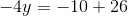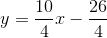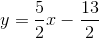Because the given line has the slope of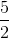, the line parallel to it must also have the same slope.

### Example Question #1 : How To Find Out If Lines Are Parallel

Linepasses through the points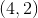and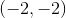. Line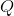passes through the point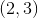and has a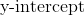of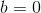. Are the two lines parallel? If so, what is their slope? If not, what are their slopes?

Yes, the lines are parallel with a slope of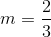.

Yes, the lines are parallel with a slope of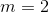.

No, the lines are not parallel. Linehas a slope ofand linehas a slope of.

No, the lines are not parallel. Linehas a slope ofand linehas slope.

Yes, the lines are parallel with a slope of.

Explanation:

Finding slope for these two lines is as easy as applying the slope formula to the points each line contains. We know that linecontains pointsand, so we can apply our slope formula directly (pay attention to negative signs!)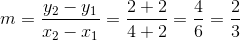.

Linecontains pointand, since the y-intercept is always on the vertical axis,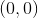. Thus: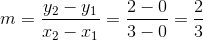The two lines have the same slope,, and are thus identical.

### Example Question #2 : How To Find Out If Lines Are Parallel

Lineis described by the equation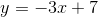. Linepasses through the points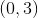and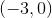. Are the two lines parallel? If so, what is their slope? If not, what are their slopes?

No, the lines are not parallel. Linehas slope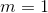and linehas slope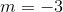.

Yes, the lines are parallel, and both lines have slope.

Yes, the lines are parallel, and both lines have slope.

No, the lines are not parallel. Linehas slopeand linehas slope.

No, the lines are not parallel. Linehas slopeand linehas slope.

Explanation:

We are told at the beginning of this problem that lineis described by. Sinceis our slope-intecept form, we can see thatfor this line. Since parallel lines have equal slopes, we must determine if linehas a slope of.

Since we know thatpasses through pointsand, we can apply our slope formula: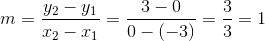Thus, the slope of lineis 1. As the two lines do not have equal slopes, the lines are not parallel.